• 最小费用流的允许边算法运用于运输问题,提出了求解运输问题的一种新解法。构造运输问题的最小费用最大流模型,并用允许边算法求得容量-费用网络的最小费用最大流,此最大流对应于运输问题的最优调运方案。在迭代过程...
• 最小费用流问题，网络分析，最大流量最小费用流问题， 支撑树等一些列方法与例题。
• 为了运用蚁群算法解决最小费用流问题,首先结合有向网络描述了最小费用流数学模型,运用从终点向始点反向计算的思想求解在最大可行流约束下的最小费用,然后给出了其具体过程。最后通过仿真实验,调整圈法和标号算法验证...
• 本文研究了无容量限制的带固定费用和可变费用的单物资和二物资的最小费用流问题,并分别给出了多项式算法。最后应用该算法,计算了一个二物资的最小费用流问题的实例。
•模糊数学
• 一、最大流问题 如下图所示，假设需要把一些物品从结点S（称为源点）运送到结点t（称为汇点），可以从其它结点中转。每条边上的权值（左图）表示该条路径最多能运送的物品数，右图边上的权值第一个数表示实际运送的...
一、最大流问题
如下图所示，假设需要把一些物品从结点S（称为源点）运送到结点t（称为汇点），可以从其它结点中转。每条边上的权值（左图）表示该条路径最多能运送的物品数，右图边上的权值第一个数表示实际运送的物品数目（flow），第二个数表示最多能运送物品的数目（即容量capacity），也称为题目中的上限。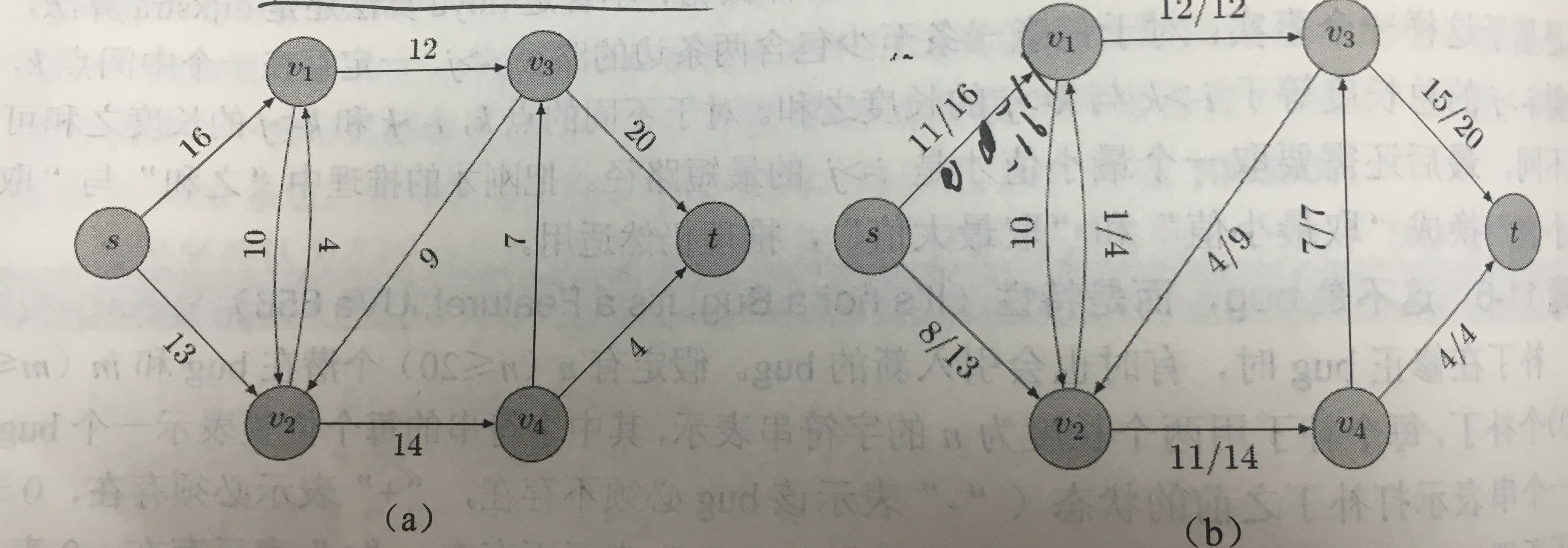最大流问题的三个性质：
（1）容量限制：f(u,v)<c(u,v)，即每条边的流量小于容量，对于不存在的边c(u,v)=0；
（2）流量平衡：对于除了s和t结点外的任意结点u,;
（3）斜对称性：f(u,v)=-f(v,u)，把3个物品从u运送到v等同于把3个物品从v运送到u，这样规定f(u,v)和f(v,u)最多只有一个正数（可以均为0）.
问题目标：
从s点流出的净流量（等于t流入的净流量）最大。
二、最大增广路算法
求解最大流问题的算法，从零流（所有边的流量均为0）开始不断增加流量，保持每次增加流量后都满足容量限制，斜对称性和流量平衡三个条件。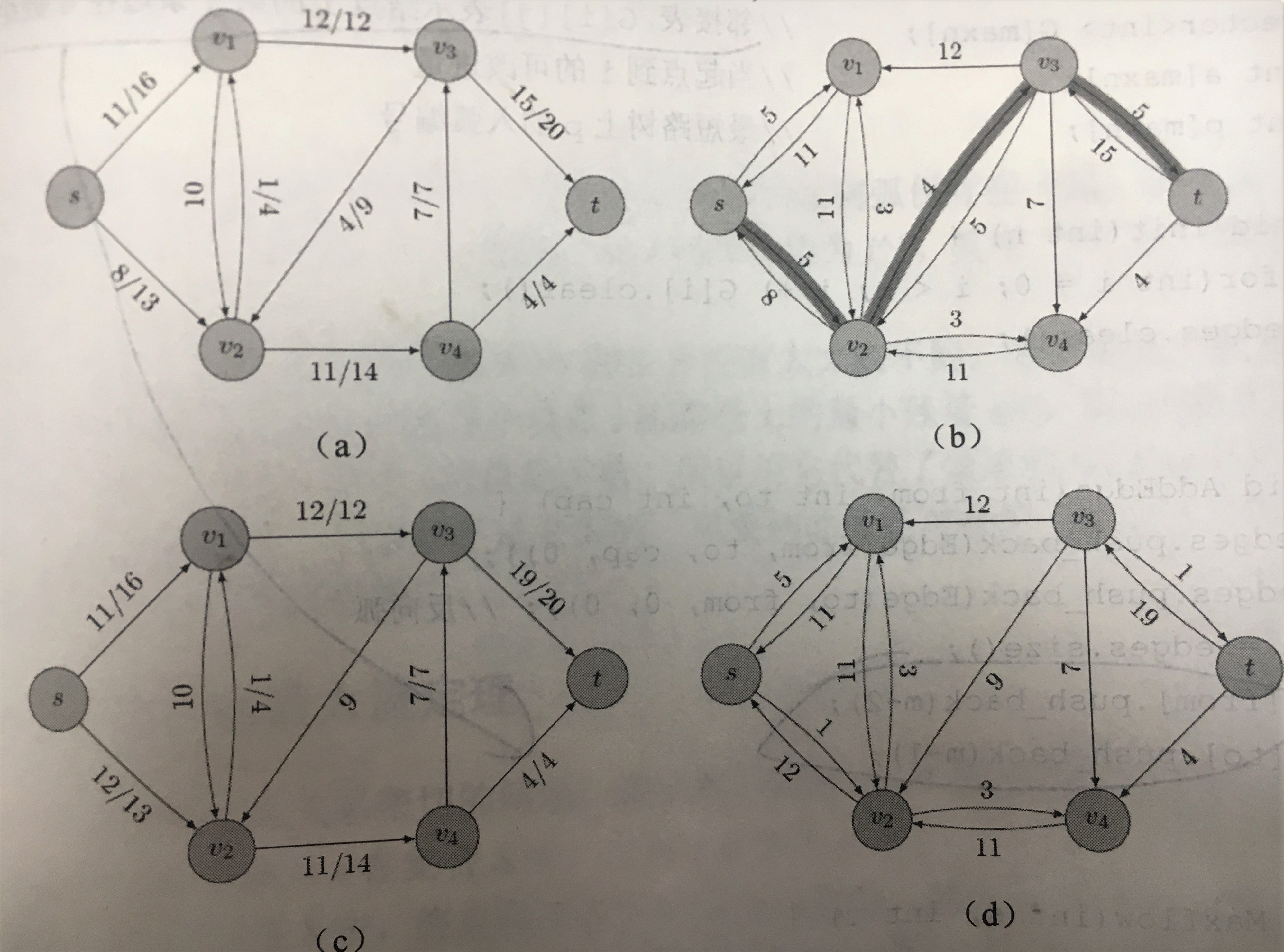如上图所示，根据图（a)中的各边值计算出每条边的容量与流量之差——残余容量，即残量。
例如
s到v2这条边：s->v2=13-8=5; v2->s=0-(-8)=8;
v2到v3这条边：v2->v3=0-(-4)=4; v3->v2=9-4=5;
注意残量网络中的边数可达到原图中边数的两倍。
算法思想：从s到t的任何一条道路都对应原图中的一条增广路（augmenting path)，只要求出该道路中所有残量的最小值d，把对应的所有边上的流量加上d即可，直到d为0不可增加为止停止迭代。
代码如下：
struct Edge
{
int from,to,cap,flow;
Edge(int a,int b,int c,int d):from(a),to(b),cap(c),flow(d){}
};
struct EdmondsKarp
{
int n,m;
vector<int> G[maxn];
vector<Edge> edges;
int a[maxn];
int p[maxn];
void init()
{
int i;
for(i=0;i<n;i++)
{
G[i].clear();
}
edges.clear();
}
{
edges.push_back(Edge(u,v,c,0));
edges.push_back(Edge(v,u,0,0));
m=edges.size();
G[u].push_back(m-2);
G[v].push_back(m-1);

}
int maxFlow(int s, int t)
{
int flow=0,i,u;
for(;;)
{
memset(a,0,sizeof(a));
queue<int> q;
q.push(s);
while(!q.empty())
{
int x=q.front();q.pop();
for(i=0;i<G[x].size();i++)
{
Edge& e=edges[G[x][i]];
if(!a[e.to]&&e.cap>e.flow)
{
p[e.to]=G[x][i];
a[e.to]=min(a[x],e.cap-e.flow);
q.push(e.to);
}
}
if(a[t]) break;
}
if(!a[t]) break;
for(u=t;u!=s;u=edges[p[u]].from)
{
edges[p[u]].flow+=a[t];
edges[p[u]^1].flow-=a[t];
}
flow+=a[t];
}
return flow;
}
};
采用BFS找增广路，a[]的值为0表示未被访问。
三。最小费用最大流问题
给网络流增加一个因素——费用cost，单位流量所需的cost。如图所示，c和a分别表示容量和费用。有图给出了一个在总流量最大的前提下，总费用最小的流。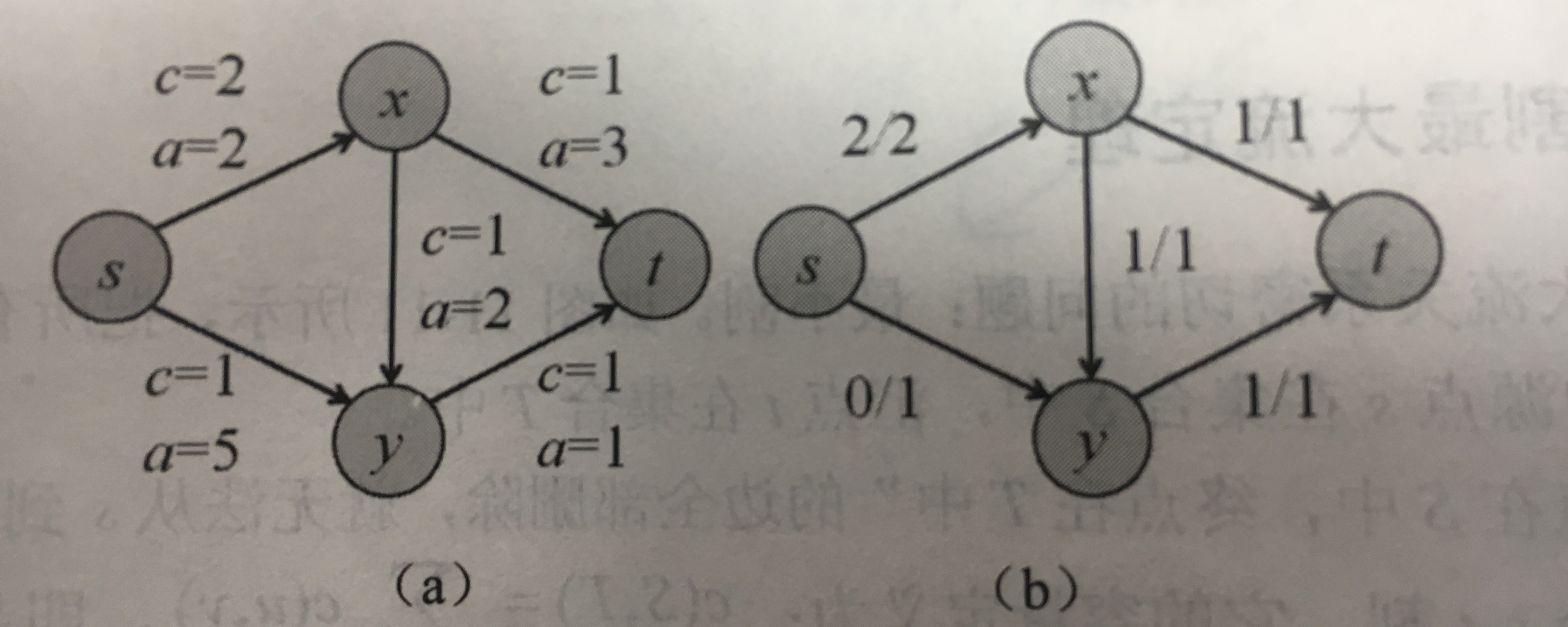在最小费用流中，平行边变得有意义了，可能会有两条边从u到v。由于费用的出现，无法合并这两条弧。先假定图中不存在平行边或反向边，用两个邻接矩阵cap和cost保存各边的容量和费用。为了允许反向增广，规定cap[v][u]=0并且cost[v][u]=-cost[u[[v]。
代码：
struct Edge
{
int from,to,cap,flow,cost;
Edge(int u,int v,int c,int f,int w):from(u),to(v),cap(c),flow(f),cost(w){}
};
struct MCMF
{
int n,m;
vector<int> G[maxn];
vector<Edge> edges;
int inq[maxn];
int d[maxn];
int p[maxn];
int a[maxn];
void init(int n)
{
this->n=n;
for(int i=0;i<n;i++)
{
G[i].clear();
}
edges.clear();
}
void addEdge(int from,int to,int cap,int cost)
{
edges.push_back(Edge(from,to,cap,0,cost));
edges.push_back(Edge(to,from,cap,0,-cost));
m=edges.size();
G[from].push_back(m-2);
G[to].push_back(m-1);
}
bool bellmanFord(int s,int t,int& flow,long long &cost)
{
for(int i=0;i<n;i++) d[i]=INF;
memset(inq,0,sizeof(inq));
d[s]=0;inq[s]=1;p[s]=0;a[s]=INF;
queue<int> q;
q.push(s);
while(!q.empty())
{
int u=q.front();q.pop();
inq[u]=0;
for(int i=0;i<G[u].size();i++)
{
Edge& e=edges[G[u][i]];
if(e.cap>e.flow&&d[e.to]>d[u]+e.cost)
{
d[e.to]=d[u]+e.cost;
p[e.to]=G[u][i];
a[e.to]=min(a[u],e.cap-e.flow);
if(!inq[e.to])
{
q.push(e.to);
inq[e.to]=1;
}
}
}
}
if(d[t]==INF) return false;
flow+=a[t];
cost+=(long long)d[t]*(long long)a[t];
for(int u=t;u!=s;u=edges[p[u]].from)
{
edges[p[u]].flow+=a[t];
edges[p[u]^1].flow-=a[t];
}
}
int MincostMaxflow(int s,int t,long long &cost)
{
int flow=0;cost=0;
while(bellmanFord(s,t,flow,cost));
return flow;
}
};
没有再采用BFS，而是BellmanFord算法寻找增广路。只要初始流是该流量下的最小费用可行流，每次增广后的新流都是新流量下的最小费用流。
展开全文算法竞赛
• ## 最小费用流及其求法

万次阅读 多人点赞 2019-05-04 08:33:48
【1】图与网络模型及方法：图与网络的基本概念 【2】图&网络模型应用—最短路径问题 【3】树：基本概念与最小生成树 ...【7】最小费用流及其求法 ： 【8】最大流问题 【10】钢管订购和运输问题 目录...

【1】图与网络模型及方法：图与网络的基本概念
【2】图&网络模型应用—最短路径问题
【3】树：基本概念与最小生成树
【4】匹配问题： 匈牙利算法 、最优指派、相等子图
【5】Euler 图和 Hamilton 图
【6】计划评审方法和关键路线法【统筹方法】：广泛地用于系统分析和项 目管理
【7】最小费用流及其求法 ：
【8】最大流问题
【10】钢管订购和运输问题

目录
1 最小费用流                         最小费用流问题的线性规划表示                例 19（最小费用最大流问题）
2 求最小费用流的一种方法—迭代法

1 最小费用流
上面我们介绍了一个网络上最短路以及最大流的算法，但是还没有考虑到网络上流 的费用问题，在许多实际问题中，费用的因素很重要。例如，在运输问题中，人们总是 希望在完成运输任务的同时，寻求一个使总的运输费用最小的运输方案。这就是下面要 介绍的最小费用流问题。
最小费用流问题的线性规划表示
在运输网络 N = (s,t,V, A,U) 中，设  是定义在 A 上的非负函数，它表示通过弧 (i, j) 单位流的费用。所谓最小费用流问题就是从发点到收点怎样以最小费用输送一已 知量为v( f ) 的总流量。 最小费用流问题可以用如下的线性规划问题描述：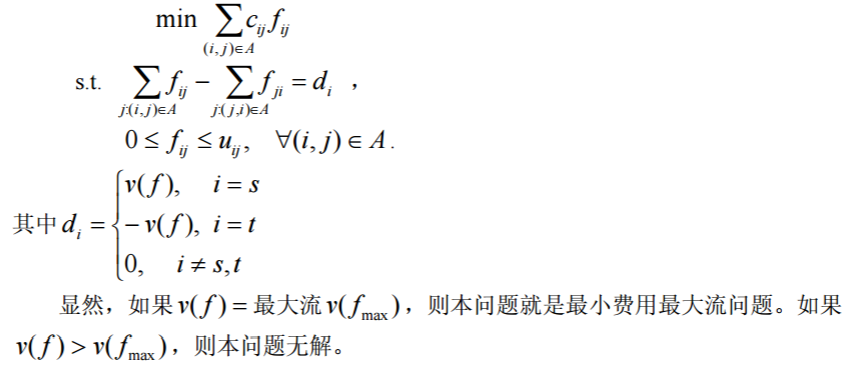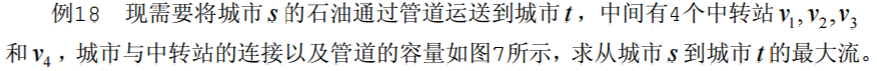例 19（最小费用最大流问题）
（续例 18）由于输油管道的长短不一或地质等原因， 使每条管道上运输费用也不相同，因此，除考虑输油管道的最大流外，还需要考虑输油 管道输送最大流的最小费用。图 8 所示是带有运费的网络，其中第 1 个数字是网络的容 量，第 2 个数字是网络的单位运费。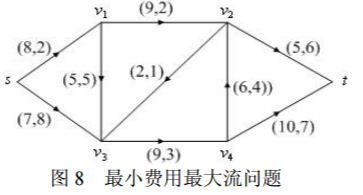解 按照最小费用流的数学规划写出相应的 LINGO 程序如下：
model:
sets:
nodes/s,1,2,3,4,t/:d;
arcs(nodes,nodes)/s 1,s 3,1 2,1 3,2 3,2 t,3 4,4 2,4 t/:c,u,f;
endsets
data:
d=14 0 0 0 0 -14; !最大流为14;
c=2 8 2 5 1 6 3 4 7;
u=8 7 9 5 2 5 9 6 10;
enddata
min=@sum(arcs:c*f);
@for(nodes(i):@sum(arcs(i,j):f(i,j))-@sum(arcs(j,i):f(j,i))=d(i));
@for(arcs:@bnd(0,f,u));
end
求得最大流的最小费用是 205，而原最大流的费用为 210 单位，原方案并不是最优 的。 类似地，可以利用赋权邻接矩阵编程求得最小费用最大流。LINGO 程序如下：
model:
sets:
nodes/s,1,2,3,4,t/:d;
arcs(nodes,nodes):c,u,f;
endsets
data:
d=14 0 0 0 0 -14;
c=0; u=0;
enddata
calc:
c(1,2)=2;c(1,4)=8;
c(2,3)=2;c(2,4)=5;
c(3,4)=1;c(3,6)=6;
c(4,5)=3;c(5,3)=4;c(5,6)=7;
u(1,2)=8;u(1,4)=7;
u(2,3)=9;u(2,4)=5;
u(3,4)=2;u(3,6)=5;
u(4,5)=9;u(5,3)=6;u(5,6)=10;
endcalc
min=@sum(arcs:c*f);
@for(nodes(i):@sum(nodes(j):f(i,j))-@sum(nodes(j):f(j,i))=d(i));
@for(arcs:@bnd(0,f,u));
end 
2 求最小费用流的一种方法—迭代法
这里所介绍的求最小费用流的方法叫做迭代法。这个方法是由 Busacker 和 Gowan 在 1961 年提出的。其主要步骤如下：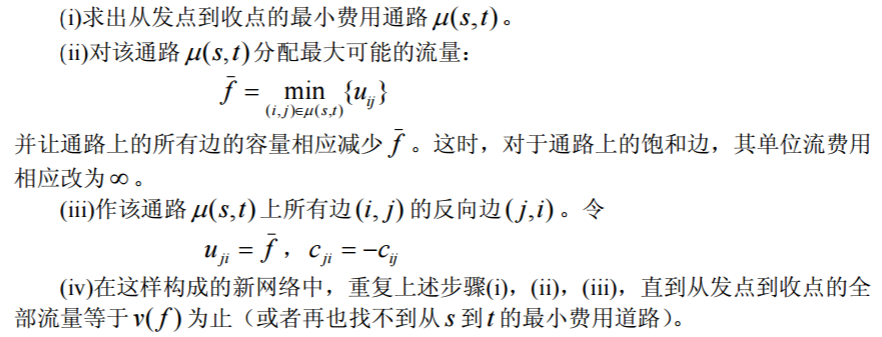下面我们编写了最小费用最大流函数 mincostmaxflow，其中调用了利用 Floyd 算法 求最短路的函数 floydpath。
求解例 19 具体程序如下（下面的全部程序放在一个文件中）：
function mainexample19
clear;clc;
global M num
c=zeros(6);u=zeros(6);
c(1,2)=2;c(1,4)=8;c(2,3)=2;c(2,4)=5;
c(3,4)=1;c(3,6)=6;c(4,5)=3;c(5,3)=4;c(5,6)=7;
u(1,2)=8;u(1,4)=7;u(2,3)=9;u(2,4)=5;
u(3,4)=2;u(3,6)=5;u(4,5)=9;u(5,3)=6;u(5,6)=10;
num=size(u,1);M=sum(sum(u))*num^2;
[f,val]=mincostmaxflow(u,c)
%求最短路径函数
function path=floydpath(w);
global M num
w=w+((w==0)-eye(num))*M;
p=zeros(num);
for k=1:num
for i=1:num
for j=1:num
if w(i,j)>w(i,k)+w(k,j)
w(i,j)=w(i,k)+w(k,j);
p(i,j)=k;
end
end
end
end
if w(1,num) ==M
path=[];
else
path=zeros(num);
s=1;t=num;m=p(s,t);
while ~isempty(m)
if m(1)
s=[s,m(1)];t=[t,t(1)];t(1)=m(1);
m(1)=[];m=[p(s(1),t(1)),m,p(s(end),t(end))];
else
path(s(1),t(1))=1;s(1)=[];m(1)=[];t(1)=[];
end
end
end
%最小费用最大流函数
function [flow,val]=mincostmaxflow(rongliang,cost,flowvalue);
%第一个参数：容量矩阵；第二个参数：费用矩阵；
%前两个参数必须在不通路处置零
%第三个参数：指定容量值（可以不写，表示求最小费用最大流）
%返回值 flow 为可行流矩阵,val 为最小费用值
global M
flow=zeros(size(rongliang));allflow=sum(flow(1,:));
if nargin<3
flowvalue=M;
end
while allflow<flowvalue
w=(flow<rongliang).*cost-((flow>0).*cost)';
path=floydpath(w);%调用 floydpath 函数
if isempty(path)
val=sum(sum(flow.*cost));
return;
end
theta=min(min(path.*(rongliang-flow)+(path.*(rongliang-flow)==0).*M));
theta=min([min(path'.*flow+(path'.*flow==0).*M),theta]);
flow=flow+(rongliang>0).*(path-path').*theta;
allflow=sum(flow(1,:));
end
val=sum(sum(flow.*cost)); 

【1】图与网络模型及方法：图与网络的基本概念
【2】图&网络模型应用—最短路径问题
【3】树：基本概念与最小生成树
【4】匹配问题： 匈牙利算法 、最优指派、相等子图
【5】Euler 图和 Hamilton 图
【6】计划评审方法和关键路线法【统筹方法】：广泛地用于系统分析和项 目管理
【7】最小费用流及其求法 ：
【8】最大流问题
【10】钢管订购和运输问题

3 图与网络模型习题
1. 一只狼、一头山羊和一箩卷心菜在河的同侧。一个摆渡人要将它们运过河去， 但由于船小，他一次只能运三者之一过河。显然，不管是狼和山羊，还是山羊和卷心菜， 都不能在无人监视的情况下留在一起。问摆渡人应怎样把它们运过河去？
2. 北京（Pe）、东京(T)、纽约(N)、墨西哥城(M)、伦敦(L)、巴黎(Pa)各城市之间的 航线距离如表 16。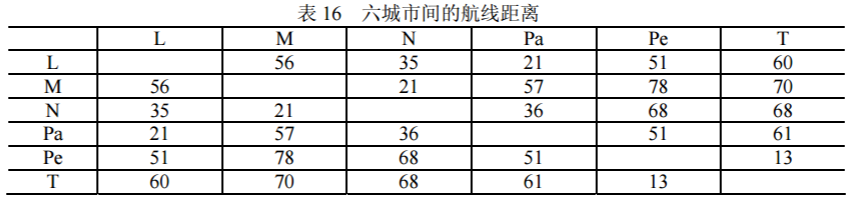由上述交通网络的数据确定最小生成树。
3. 某台机器可连续工作 4 年，也可于每年末卖掉，换一台新的。已知于各年初购 置一台新机器的价格及不同役龄机器年末的的处理价如表 17 所示。又新机器第一年运 行及维修费为 0.3 万元，使用 1-3 年后机器每年的运行及维修费用分别为 0.8，1.5，2.0 万元。试确定该机器的最优更新策略，使 4 年内用于更换、购买及运行维修的总费用为最省。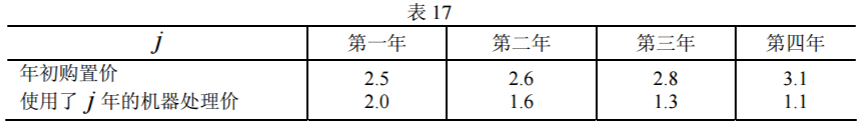4. 某产品从仓库运往市场销售。已知各仓库的可供量、各市场需求量及从i 仓库 至 j 市场的路径的运输能力如表 18 所示（表中数字 0 代表无路可通），试求从仓库可运 往市场的最大流量，各市场需求能否满足？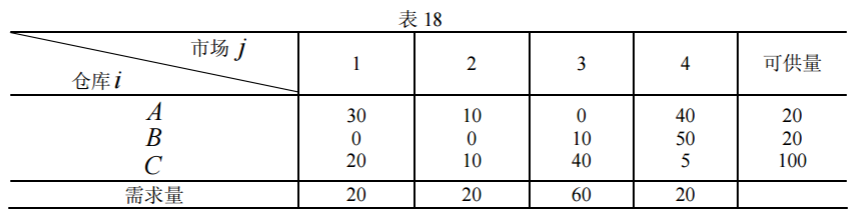5. 某单位招收懂俄、英、日、德、法文的翻译各一人，有 5 人应聘。已知乙懂俄 文，甲、乙、丙、丁懂英文，甲、丙、丁懂日文，乙、戊懂德文，戊懂法文，问这 5 个人是否都能得到聘书？最多几个得到聘书，招聘后每人从事哪一方面翻译工作？
6. 表 19 给出某运输问题的产销平衡表与单位运价表。将此问题转化为最小费用最 大流问题，画出网络图并求数值解。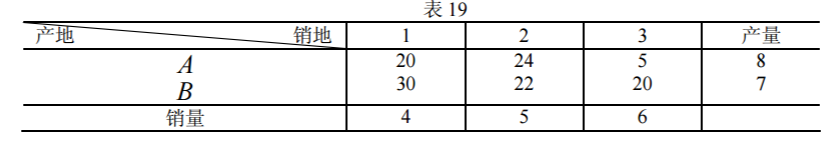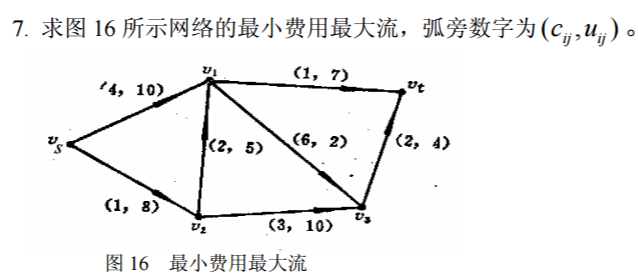8. 某公司计划推出一种新型产品，需要完成的作业由表 20 所示。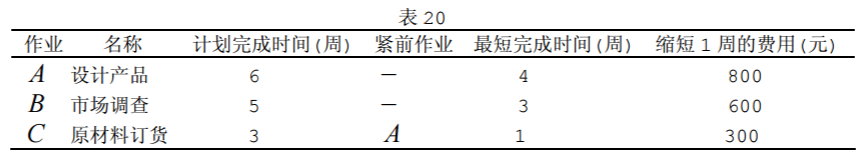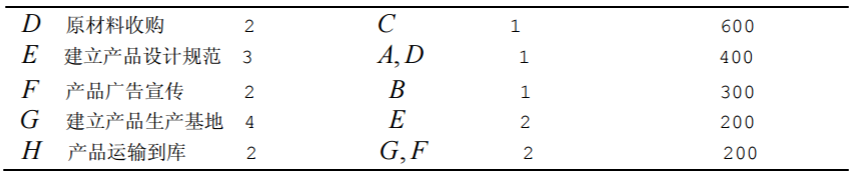（1）画出产品的计划网络图；
（2）求完成新产品的最短时间，列出各项作业的最早开始时间、最迟开始时间和 计划网络的关键路线；
（3）假定公司计划在 17 周内推出该产品，各项作业的最短时间和缩短 1 周的费 用如上表所示，求产品在 17 周内上市的最小费用；
（4）如果各项作业的完成时间并不能完全确定，而是根据以往的经验估计出来的， 其估计值如表 21 所示。试计算出产品在 21 周内上市的概率和以 95％的概率完成新产 品上市所需的周数。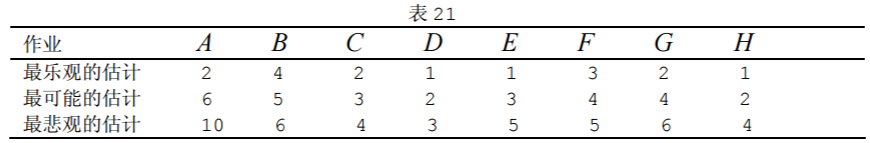9．已知下列网络图有关数据如表 22，设间接费用为 15 元/天，求最低成本日程。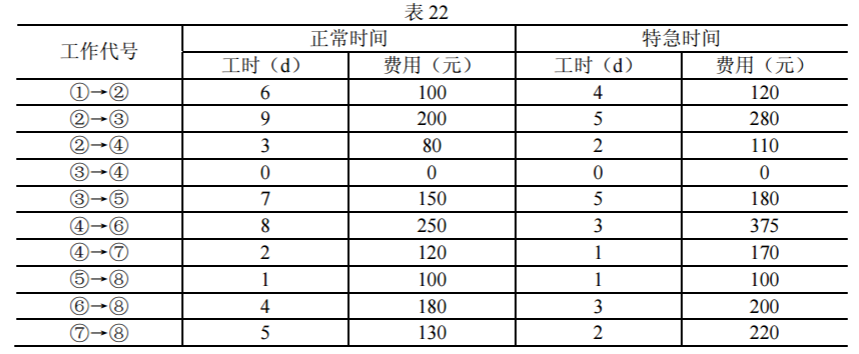展开全文• 最小费用流的最小费用路算法，运算正确返回0，若有负圈返回其中的一个节点
• 　在实际网络问题中，不仅考虑从 Vs 到 Vt 的流量最大，还要考虑可行流在网络传送过程中的费用问题，这就是网络的最小费用最大流问题。 　最小费用最大流问题的一般提法：已知容量网络 D=(V ,A ,C)，每条弧 (Vi，Vj...

复杂网络中，单源单点的最小费用最大流算法(MCMF)应用广泛。
在实际网络问题中，不仅考虑从 Vs 到 Vt 的流量最大，还要考虑可行流在网络传送过程中的费用问题，这就是网络的最小费用最大流问题。
最小费用最大流问题的一般提法：已知容量网络 D=(V ,A ,C)，每条弧 (Vi，Vj) 除了已给出容量 Cij 外，还给出单位流量的传输费用 bij≥0，记作D=(V, A, C, B)，其中bij ∈B。要在费用、容量网络 D 中寻找 Vs→Vt的最大流 f={fij}，且使流的总传输费用：
b(f)=Σbij fij 最小
从上一讲可知，最大流的求法就是在容量网络上从某个可行流出发，设法找到一条从 Vs→Vt 的增广链，然后沿着此增广链调整流量，作出新的流量增大了的可行流。在这个新的可行流基础上再寻找它的增广链。如此反复进行，直至再找不出增广链，就得到了该网络的最大流。

例子：给定费用、容量网络图(bij，cij)，试求网络的最小费用最大流。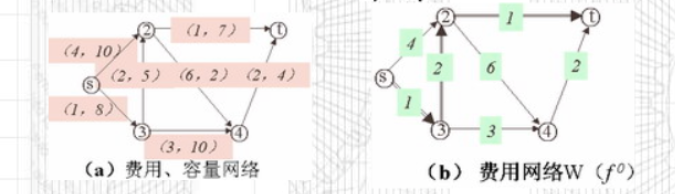解：
（1）、初始取0流量，此时总费用为 f(0) = 0。
（2）、由原始网络构建费用网络图（费用网络图每条线路上的权重为bij，bij为单位流量的费用）。
（3）、通过当前费用网络图找到一个费用最少的路径，即vs->v3->v2->vt。通过该路径上每条线段的容量得出该线路能通过的最大流量，即调整量θ = min{8,5,7} = 5，即流量为5，当前的最小费用为 f(1) = 5*1+5*2+5*1 = 20。下图为流量网络图。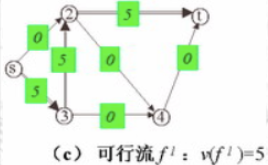（4）、在（3）的基础上构造新的费用网络图，构造方法为：当线路没流量通过时，流量方向不变，费用为原始费用。如vs->v2；
当线路流量等于该线路的最大容量时，则流量方向改变，费用为原始费用的负数。如v3->v2变为v2->v3；
当线路流量小于该线路的最大容量时，则增加反向流量，费用为原始费用的负数。如vs->v3新增v3->vs；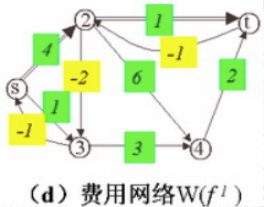（5）、在（4）中得到的费用网络图上，找到费用最少的路径，即vs->v2->vt，通过该路径上每条线段的容量得出该线路能通过的最大流量，即调整量θ = min{10,7-5} = 2，得到的最小费用流的流量为7，当前的最小费用为 f(2) = 2*4+5*1+5*2+7*1 = 30。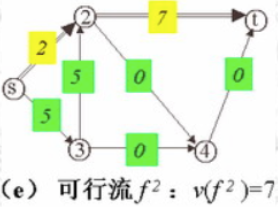（6）、构造可行流 f2的费用网络图。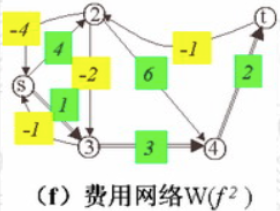（7）、在（6）中得到的费用网络图上，找到费用最少的路径，即vs->v3->v4->vt，通过该路径上每条线段的容量得出该线路能通过的最大流量，即调整量θ = min{8-5,10,4} = 3，得到的最小费用流的流量为10，当前的最小费用为 f(3) = 2*4+8*1+5*2+7*1+3*3+3*2 = 42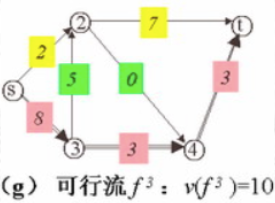（8）、构造可行流 f3 的费用网络图。（9）、在（8）的费用网络图上，找到费用最少的路径，即vs->v2->v3->v4->vt，通过该路径上每条线段的容量得出该线路能通过的最大流量，即调整量θ = min{10-2, 10-3, 4-3, 5} = 1，得到的最小费用流的流量为11，当前最小费用为 f(4) = 3*4+8*1+4*2+7*1+4*3+4*2 = 55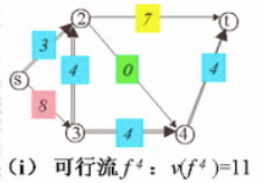（10）、构造可行流 f4 的费用网络图。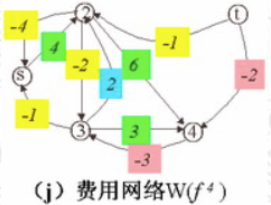由于无法找到从 vs->vt 的最短路，所以 f(4) 就是该网络的最小费用最大流。

转载于:https://www.cnblogs.com/keye/p/11059148.html
展开全文• 网络流之最小费用流问题(不完善)
简介
最小费用流问题就是一个最小费用流是指从带权图的起点到终点的路径,其权值之和最小。
具体做法
1.首先构造一个图，这个图的每一条边有一个容量c，有一个权值v。  2.再按照v来跑遍spfa求最短路径，并将这条最短路径记录下来。(设pre[i]=j表示在这条最短路径中，i是从j走过来的。每当可以更新最短路径时就可以同时更新pre啦~)  这个时候判断一下dis[t]是否为∞，如果是，则说明找不到增广路径，算法结束；否则说明找到增广路径，继续。  3.从汇点t开始往回倒，记录

min c(i,j)=m
$min\ c(i,j)=m$，统计

Σv(i,j)
$\Sigma v(i,j)$  4.在从t开始，往回倒一次，这次

c(i,j)−=m,c(j,i)+=m
$c(i,j)-=m,c(j,i)+=m$，然后转2.
由于本人弱，不会严格证明。
一个Code
这串代码类似于模板，  题目：n个盒子被放成一圈，每个盒子按顺时针编号为1到n，(1<=n<=1000)。每个盒子里都有一些球，且所有盒子里球的总数不超过n。  这些球要按如下的方式转移：每一步可以将一个球从盒子中取出，放入一个相邻的盒子中。目标是使所有的盒子中球的个数都不超过1。  题解  源点与每个盒子连一条容量为盒子中球的数量，费用为0的边；  每个盒子与汇点连一条容量为1，费用为0的边；  每个盒子与左边的盒子连一条边，与右边的盒子连一条边，这些边都是容量为∞，费用为1的边。  然后跑一遍最小费用流即可。
#include<iostream>
#include<cstdio>
#include<cmath>
#include<cstring>
#define N 1005
#define M 10003
#define LL long long
#define fo(i,a,b) for(i=a;i<=b;i++)
using namespace std;
int tot,i,j,k,l,n,ans;
int pre[N],dis[N],qu[M];
int a[N];
bool bz[N];
void lb(int x,int y,int z,int w)
{
go[++tot]=y;
flow[tot]=z;
val[tot]=w;
go[++tot]=x;
flow[tot]=0;
val[tot]=-w;
}
bool spfa()
{
memset(dis,127,sizeof(dis));dis=0;
memset(bz,0,sizeof(bz));
memset(pre,0,sizeof(pre));
int t=0,w=1,i,j,now,to;
qu=0;
while (t<w)
{
now=qu[++t];
edge(i,now)
{
to=go[i];
if (flow[i]>0 && dis[to]>dis[now]+val[i])
{
dis[to]=dis[now]+val[i];
pre[to]=i;
if (!bz[to])
{
bz[to]=1;
qu[++w]=to;
}
}
}
bz[now]=0;
}
if (dis[n+1]!=2139062143) return 1;else return 0;
}
void find()
{
int x=n+1,sum=0,minc=2147483647;
while (x)
{
minc=min(minc,flow[pre[x]]);
x=go[pre[x]^1];
}
x=n+1;
while (x)
{
flow[pre[x]]-=minc;
flow[pre[x]^1]+=minc;
sum+=val[pre[x]];
x=go[pre[x]^1];
}
ans+=sum*minc;
}
int main()
{
scanf("%d",&n);
tot=1;
fo(i,1,n)
{
scanf("%d",&a[i]);
lb(0,i,a[i],0);
lb(i,n+1,1,0);
if (i>1) lb(i,i-1,2147483647,1);
if (i<n) lb(i,i+1,2147483647,1);
}
lb(1,n,2147483647,1);
lb(n,1,2147483647,1);
while (spfa()) find();
printf("%d",ans);
}
展开全文• 最小费用流问题，可视为一般化的最短路径问题和最大流问题，即只要选定合适的权重、容量、流量，解决最小费用流的方法就能用来解决上述问题。另一方面，也意味着解最小费用流问题至少和解最短路径或最大流问题一样难...算法 图算法 Floyd
• 联合大学 实验报告 项目名称 运筹学专题实验报告 学 院 自动化 专 业 物流工程 班 级 1201B 学 号81 姓 名 管水城 成 绩 2015 年 5 月 6 日 实验三使用matlab求解最小费用最大问题 一实验目的 (1)使学生在程序...
• 博文链接https://blog.csdn.net/weixin_43428682/article/details/94600364。 正确代码链接...这个代码有一点问题，不需要下载。查看我的博客中提供的链接，那个好用。适用于2014a以后的版本
• 在不同解包裹算法中,最小费用流(MCF)解包裹法可以限制残差点误差远程扩散,并将误差优先限制在低相干区域,有利于保证高相干区域解包裹结果不受干扰,精度较高,但残差点数量较多时计算效率很低。为缩短解包裹时间,提出...
• 一、 使用spfa算法解决最小费用流问题 1. 算法原理 实际是用队列优化的Bellman-ford算法，可以允许负边权的存在。SPFA算法通过维护一个队列，使得一个节点的当前最短路径被更新之后没有必要立刻去更新其他的节点...
• Bellman-Ford、SPFA、改进的Dijkstra三种算法解决最小费用最大流问题以及相关代码的实现。
• 最小费用流的负回路算法，是先任意分配流量v0，再将流量调整到权值较小的边上，参考： 基于Floyd算法的最小费用流的负回路算法（图解） 而最小费用流的最短路径算法，则是从0流开始，往最短路径上分配流量，直到流量...单源最短路径
• 最小费用流的消圈算法，判断一个费用流是否最优的消圈算法
• 基于matlab2016的最小费用最大流问题求解，内含增广链路函数[path,value] = AugmentingPath(G,s,t)和一个demo函数。 寻找增广链路时，使用了matlab自带的最短路径shortestpath函数，demo中使用了matlab自带的...
• 实 验 三 使 用 ma t l a b 求 解 最 小 费 用 最 大 问题 精品文档 北京联合大学 实验报告 项目...5 月 6 日 收集于网络如有侵权请联系管理员删除 精品文档 实验三使用 matlab 求解最小费用最大问题 一实
• 费用流问题。 【建模方法】 把所有仓库看做二分图中顶点Xi，所有零售商店看做二分图中顶点Yi，建立附加源S汇T。 1、从S向每个Xi连一条容量为仓库中货物数量ai，费用为0的有向边。 2、从每个Yi向T连一条容量为商店所...
• ## Java实现最小费用最大流问题

万次阅读 多人点赞 2019-07-26 17:48:33
在最大有多组解时，给每条边在附上一个单位费用的量，问在满足最大时的最小费用是多少？ 2 解决方案 下面代码所使用的测试数据如下图： package com.liuzhen.practice; import java.util.ArrayList; import ...Java 最大流
• ## 最小费用最大流(详解+模板)

万次阅读 多人点赞 2018-01-19 18:49:17
By Bartholomew By Bartholomew SPFA完整代码： 重点代码：Primal-Dual 原始对偶算法...∴ 最小费用流的充要条件 ＜=＞ 剩余网络之中没有 负环 性质2: 性质3: 先感谢大家提出来的宝贵建议 （Than...
• 实验三使用matlab求解最小费用最大问题 精品文档 精品文档 收集于网络如有侵权请联系管理员删除 收集于网络如有侵权请联系管理员删除 精品文档 收集于网络如有侵权请联系管理员删除 北京联合大学 实验报告 项目...
• 在网上翻了很久，终于找到一个大概看得懂的证明了。 https://bartholomewa.wordpress.com/2018/04/25/最小费用最大详解模板/
• Description  W公司有m个仓库和n 个零售商店。...试设计一个将仓库中所有货物运送到零售商店的运输方案，使总运输费用最少。  对于给定的m 个仓库和n 个零售商店间运送货物的费用，计算最优运输方案和最差运输方案。acm...